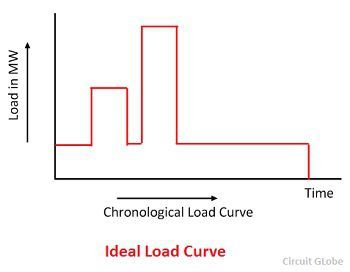Definition: Load curve or chronological curve is the graphical representation of load (in kW or MW) in proper time sequence and the time in hours. It shows the variation of load on the power station.When the load curve is plotted for 24 hours a day, then it is called daily load curve. If the one year is considered then, it is called annual load curve.

The load curve of the power system is not same all the day. It differs from day to day and season to season. The load curve is mainly classified into two types, i.e., the summer load curve and the winter load curve.## Information Obtained From Load Curve

The following are the information obtained from load curves.

1. Load duration curve determines the load variation during different hours of the day.
2. It indicates the peak load which determines the maximum demand on the power station.
3. The area under the load curve gives the total energy generated in the period under consideration.
4. The area under the curve divided by the total numbers of hours gives the load.
5. The ratio of the area under the load curve of the total area of the rectangle in which it is contained gives the load factor.

The ideal load curve is flat, but practically it is far from flat. For a flat load curve, the load factor will be higher. Higher load factor means the more uniform load pattern with fewer variations in load.

###Utility of Load Curve

The following are the utility of the load curve.

1. Load curve decides the installed capacity of a power station.
2.  It is helpful in choosing the most economical sizes of the various generating units.
3. The load curve estimates the generating cost.
4. It decides the operating schedules of the power station, i.e., the sequence in which the different generating units should run.